# Phi's Fascinating Figures

On this page we meet some more marvellous maths about the number Phi itself, its multiples and powers.
The calculators on this page require JavaScript. If JavaScript is enabled then this panel will disappear in a few seconds. If you have switched JavaScript off (if it is disabled) then please go to the Preferences for this browser and enable it if you want to use the calculators, then Reload this page.
The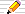icon means there is a You do the maths... section of questions to start your own investigations. The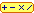calculator icon indicates that there is a live interactive calculator in that section.

1·61803 39887 49894 84820 45868 34365 63811 77203 09179 80576 ..More..## Powers of Phi

We return to the definition of Phi, as the positive valued solution to
P(P-1)=1
P here is either of 2 values, Phi and 1-Phi.
So P has the property that P2=P+1.
Let's look at Phi2 first.

### Phi squared

We can read the equation above as to find P2, just add 1 to P.

#### You do the maths...1. Use your calculator to evaluate Phi=(1+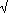5)/2. If you have a Memory on your calculator, store this value in it.
2. Square it and check that it is just Phi+1 (i.e. subtract one and compare with the Memory value).
3. Use your calculator to evaluate the other value (1-Phi) or (1-Memory) = (1-5)/2
4. Again square the value just found and check that it is just the same as adding 1 to the value you squared.
Now let's look at Phi3.

### Phi cubed

Is there another way to calculate Phi3 apart from just Phi x Phi x Phi?

Yes - let's see how to compute it in two more ways. We use the basic P(P-1)=1 formula or, in another form, P2=P+1.
P3 is just P.P2 = P(P+1) by our "basic formula", which expands to P2+P

...now that's interesting!... 1 + P = P2 AND P + P2 = P3 ...hmmm! Is there anything in this do you think?
Question: How could this be generalized? We'll use this result in the next sub-section about a Phi-bonacci Brick... but, for now, let's get back to the original equation...
and P2 + P = (P+1) + P after using the "basic formula" again and this is just 2P + 1. So
Phi3 = 1 + 2 Phi
Notice that this needs just one multiplication rather than two if we evaluated Phi x Phi x Phi. That's the first quick way.

The second answer was spotted by Scott Beach but it is also in the table at the foot of this page: since Phi=(√5+1)/2 then 2Phi is √5+1 and 1+ 2 Phi = &radic 5 + 2:

Phi3 = 2 + √5

#### Interesting Facts about a Phi-bonacci Brick

[This variation was suggested by Hud Nordin of Sunnyvale, California.]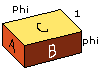If we have a brick with sides of lengths 1, Phi=1·61803... and phi=1/Phi=0·61803... then:-
• the longest side is the sum of the other two lengths since 1 + phi = Phi
• the largest face (area C=1 x Phi) is the sum of the other two face's areas (area A =1 x phi and area B=phi x Phi) since
Area A + Area B
= 1 x phi + phi x Phi
= phi + 1
= Phi
= Area C
The next three interesting facts and figures on the Phibonacci brick were first pointed out by Donald Seitz in The Mathematics Teacher, 1986, pages 340-341 in an article entitled A Geometric Figure Relating the Golden Ratio to Pi.
• What is the surface area S of the brick?
Above we saw that the sum of the 2 smaller face's areas equals the largest face's area, and that this is Phi.
Since there are 2 faces with smallest area, 2 of middle-sized area (which total 2 times the largest face area, that is 2 Phi) and we also have two other faces of the largest area (Phi) , then:
The surface area of the brick is 4 Phi
• How long is the diagonal across the brick?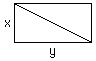Another surprise awaits us when we calculate the length of the diagonal across the brick.
The formula is a 3-dimensional analogue of Pythagoras Theorem.
For a rectangle of sides x and y, its diagonal is(x2 + y2).
For a 3-D brick with sides x,y and z, its diagonal has length(x2 + y2 + z2).

So how long is the diagonal of our Phi-bonacci brick? Since its sides (x,y and z) are 1, Phi and phi, the length of its diagonal is:(12 + Phi2 + phi2). I'll leave you to check the algebra but the surprisingly simple answer is

The diagonal of the brick has length 2
• A relationship between Phi and PiEven more surprising is that the brick shows a simple relationship between Pi and Phi, using the values for the diagonal D that we have just found and the surface area S.
If we imagine the brick tightly packed into a sphere, the centre of the sphere will be half way along diagonal D.
So the radius of the sphere will be 1 and its surface area will be 4 Pi.

We showed above that the surface area of the brick, S, is 4 Phi.
Putting these two together we have:

The ratio of the surface areas of
the Phibonacci brick and its surrounding sphere
is Phi : Pi

#### You do the maths...1. Suppose we have a brick with sides a, b and c. Suppose also that the sum of the smaller sides equals the longest side (i.e. that a+b=c). Also suppose we have the "Area sum property" too, that the smaller two areas add up to the larger, or that ab + ac = bc. The questions here show that the only way to have BOTH properties is when a and b as well as b and c are in the golden ratio (Phi):- Show that, if we let k stand for a/b then we must also have k = b/c .
2. Show that k must also satisfy k2 = 1 + k
3. ... and hence show that k must be Phi.
• Golden Triangles, Rectangles and Cuboids by M Bicknell and V E Hoggatt Jr in Fibonacci Quarterly vol 7 (1969), pages 73-91 is a very readable account of some generalizations of these results.

### The Golden rectangle and powers of phi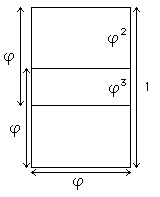On a golden rectangle with sides of length phi and 1, dividing at the golden ratio point gives two overlapping squares whose sides are phi.
The gap between a square and the longer side has length 1-phi which is also phi2.
So the phi by phi squares are themselves divided at their own golden section ratios by the side of the other overlapping square. The size of the overlap is phi-phi2 which is just phi3 (because the equation 1-phi=phi2 can be multiplied by phi to give the relation phi-phi2 = phi3).

As an exercise, how would you easily construct a line of length phi4 = phi2-phi3 on the diagram?

### Higher powers of phi

Here is another geometrical illustration of phi2, phi3 and phi4: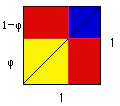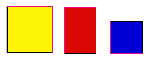If we take a square with sides of length 1 and divide two sides at the golden section point, we form two new squares (yellow and blue) and two (red) rectangles. Notice that the diagonal is also divided at its golden section point too.
The large yellow square has sides of length phi, so its area is phi2.
The sides of a red rectangle are of length phi and 1-phi=phi2 so each has an area of phi3!
The small blue square has sides of length 1-phi=phi2 so its area is phi4!!
So, the squares plus the rectangles area must add up to 1, the area of the big square and we have yellow + 2 blue + red = 1:
1 = phi2 + 2 phi3 + phi4

#### You do the maths...1. Evaluate Phi and raise it to the power 4 (hint: square it twice) on your calculator. Save this value in a memory (or write it down).
2. Compare the value you have just found with (3 Phi+2).
3. What is Phi5 in the form a Phi + b (ie find the integers a and b) ?
4. Write Phi6 in the same form.
5. Can you spot a general pattern for Phin? Hint: spot the well-known series for the values of a and b in the successive powers of Phi?

6.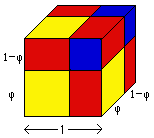Here is a cube with sides divided at the golden section points to split it into eight pieces. The eight pieces are of 4 different shapes: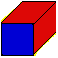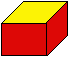There are 8 blocks in the cube: how many of each shape are there?
What is the volume of each? ( Each volume will be a power of phi!)
If the total volume is 1 (since the original cube has sides of length 1), what relationship does this suggest between your multiples of powers of phi and 1?
How is this answer related to the relationship you found in the last question?

### A relation on Powers of Phi

There's an even more intriguing relationship between the powers of Phi than the one you discovered for yourself in the last section. Here's how you can find it:

#### You do the maths...1. What do you notice about the difference between Phi3 and Phi2?
2. and how does Phi4-Phi3 compare with another power of Phi?
3. Can you guess what we must add to Phi4 to get Phi5.
4. What is the general pattern:
Phin = Phin-1 + ?
5. Can you justify this using your answer to the last section? that is, using your pattern for
Phin = a Phi + b

1·61803 39887 49894 84820 45868 34365 63811 77203 09179 80576 ..More..## Multiples of Phi

We've just seen that we can use multiples of Phi to calculate its powers easily and you might think that there's not much else we can discover about multiples of Phi.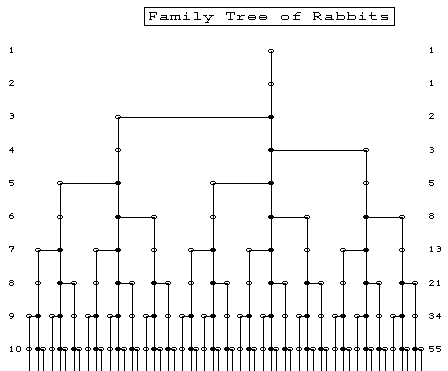Here we show another relationship and also explain how the Rabbits family Tree diagram was made, originally seen in the Fibonacci and Nature. It's all to do with the multiples of Phi, or, rather, the fractional parts of the multiples.

### You do the maths...1. On a piece of paper, draw a line 10cm long near the top, or use graph paper. This is going to be used to plot the fractional parts of the multiples of Phi=1·618.. so it will represent values from 0 to 1 (0·5 being at 5cm, 1 at 10cm etc).
2. Draw the first point at the fractional part of Phi itself=0.618, ie at 6·18 cm along the line. Mark it 1, since we used 1 times Phi.
3. Draw another line underneath the first. On it put a point for the fractional part of 2Phi=3.236 ie at 2·36cm. Mark the point as 2.
4. Draw another line underneath the last. On it put a point for the fractional part of 3Phi=4.854, ie at 8·54cm. Mark it as 3.
5. Draw a new line and this time mark TWO points labelled 4 and 5,
so that this line will have the next 2 multiples of Phi: 4Phi and 5Phi.
6. The next line will have the next THREE multiples of Phi: 6Phi, 7Phi and 8Phi.
7. The next line will have the next FIVE multiples of Phi.
8. The next line will have the next EIGHT multiples of Phi.
9. ..you can guess the next one!
10. When you're tired of drawing lines and plotting points, add some thick vertical lines down from each point.
11. Where each vertical line begins, draw a thick horizontal line to the nearest vertical line.

We can interpret the thick lines of the Rabbit Family Tree as follows:

1. The 10 cm lines across the page each represent one new generation of rabbits, or each new month in Fibonacci's original problem.
2. Each thick vertical line represents one of our rabbit pairs and..
3. .. each pair is "born" when it appears for the first time as a branch from an thick vertical line that started higher up the page (its "parents").
4. Notice how each vertical line goes through two levels (generations or months) before it starts to branch and ..
5. .. then it branches on every line after that. This shows where our rabbits must be 2 months old to start producing their own young and then do so every month after that.
6. Notice how on each generation level, the new rabbits appear equally and optimally spaced across the page so that there is no overcrowding at one point in the tree...
7. .. and also that each horizontal branching line is the same length on each generation and ...
8. .. all are on the same side of their "parents" line in each generation and...
9. ..the side they appear on swops each time, from left side to right side to left.
You can probably spot some more relationships in this Tree (for instance, that the lengths of the horizontal branches are each 0·618 times shorter than those of the previous generation).

1·61803 39887 49894 84820 45868 34365 63811 77203 09179 80576 ..More..## Continued Fraction representations

A continued fraction is a fraction of the form:
```    a +            1            = a + 1/( b + 1/( c + 1/( d + ... )))
b +        1
c +    1
d + ...
```
where a, b, c etc are integers (often only positive).
Phi is the simplest form of continued fraction since all the integers a, b, c, ... are 1.
Writing such fractions in the usual form, as above, takes a lot of space on the page, so an abbreviation often used is to just give the integers as a list: [a,b,c,...].
```    a +            1            = [ a, b, c, d, ... ]
b +        1
c +    1
d + ...
```
In this notation then Phi=[1,1,1,1...] which we can further abbreviate to  where the numbers shown like this indicate that they are repeated in the same cycle for ever. In some books, these periodic parts may have dots over them in the same way that we write infinite decimal fractions such as
 . . . . 1/3 =0·3333.. =0· 3 ;    1/7 = 0· 1 4285 7 = 0·142857142857142...;     1/12 = 0·0833333... = 0·08 3

and other authors use an overline like this so that 1/7=0·142867.
If we have more than one integer in the repeating part, such as [6,1,5,1,5,1,5,...] we write [6,1,5].

For more see Introduction to Continued Fractions at this site.

1·61803 39887 49894 84820 45868 34365 63811 77203 09179 80576 ..More..## Formulae for Phi

Phi denotes (5+1)/2 =1·6180339... and
phi denotes (5-1)/2 =0·6180339... .

The basic formulae are

Phi = 1/phi = phi + 1
Phi2 = Phi + 1 and therefore
phi2 = 1 − phi.

These can be generalized and give an important and remarkable pair of results for all integers n (positive, zero and negative):

 Phin-2 + Phin-1 = Phin phin = phin+1 + phin+2

Other relationships between the powers of Phi and phi and the Fibonacci numbers that also hold for all integer values of n are:

Main = Other = X + Y Phi = A + B phi = C + D √5
Phin = phi−n = F(n−1) + F(n) Phi = F(n+1) + F(n) phi L(n) + F(n)√5
2
(−phi)n = (−Phi)−n = F(n+1) − F(n) Phi = F(n-1) − F(n) phi L(n) − F(n)√5
2
where L(n) is the nth Lucas number, defined as L(n) = F(n+1) + F(n-1).
With thanks to John McNamara. There is a comprehensive list of these and other kinds of formlae on my Fibonacci, Phi and Lucas numbers Formulae page at this site.

###Powers and Multiples of Phi Calculator

C A L C U L A T O R
 powers multiples of Phiphi from up to
R E S U L T S

### Numerical Relationships between Phi and its powers

Summarising the above, we can make a table where we relate the powers of Phi to its multiples - and we note the Fibonacci numbers appearing again! We can also expand the multiples of Phi using its definition as (5+1)/2 and find Fibonacci again. Finally, we give the continued fraction representations of these powers with the repeating period part (if any) shown like this. In both the5 forms and the continued fractions forms we note that a new series of numbers appears - - the Lucas numbers 2, 1, 3, 4, 7, 11, 18, 29, ... .
Phi
power
phi
power
A + B PhiC + D phiKsqrt(5)+L decimal
value
continued
fraction
...
Phi9 phi−9 21 + 34 Phi 55 + 34 phi 38 + 17√5 76·0131556174.. 
Phi8 phi−8 13 + 21 Phi 34 + 21 phi (47 + 21√5)/2 46·9787137637.. [46, 1, 45]
Phi7 phi−7 8 + 13 Phi 21 + 13 phi (29 + 13√5)/2 29·0344418537.. 
Phi6 phi−6 5 + 8 Phi 13 + 8 phi 9 + 4√5 17·9442719099.. [17, 1, 16]
Phi5 phi−5 3 + 5 Phi 8 + 5 phi (11 + 5√5)/2 11·0901699437.. 
Phi4 phi−4 2 + 3 Phi 5 + 3 phi (7 + 3√5)/2 6·8541019662.. [6, 1, 5]
Phi3 phi−3 1 + 2 Phi 3 + 2 phi 2 + √5 4·2360679774.. 
Phi2 phi−2 1 + 1 Phi 2 + 1 phi (3 + √5)/2 2·6180339887.. [2, 1]
Phi1 phi−1 0 + 1 Phi 1 + 1 phi (1 + √5)/2 1·6180339887.. 
Phi0 phi0 1 1 1 1·0000000000.. 
Phi−1 phi1 −1 + 1 Phi 0 + 1 phi (−1 + √5)/2 0·6180339887.. [0, 1]
Phi−2 phi2 2 − 1 Phi 1 − 1 phi (3 − √5)/2 0·3819660112.. [0, 2, 1]
Phi−3 phi3 −3 + 2 Phi −1 + 2 phi −2 + √5 0·2360679774.. [0, 4]
Phi−4 phi4 5 − 3 Phi 2 − 3 phi (7 − 3√5)/2 0·1458980337.. [0, 6, 1, 5]
Phi−5 phi5 −8 + 5 Phi −3 + 5 phi (−11 + 5√5)/2 0·0901699437.. [0, 11]
Phi−6 phi6 13 − 8 Phi 5 − 8 phi 9 − 4√5 0·0557280900.. [0, 17, 1, 16]
Phi−7 phi7 −21 + 13 Phi −8 + 13 phi (−29 + 13√5)/2 0·0344418537.. [0, 29]
Phi−8 phi8 34 − 21 Phi 13 − 21 phi (47 − 21√5)/2 0·0212862362.. [0, 46, 1, 45]
Phi−9 phi9 -55 + 34 Phi -21 + 34 phi -38 + 17√5 0·0131556174.. [0, 76]
...
phi = 1/Phi so the powers of phi are the negative powers of Phi.
Note that [0,a,b,c,...] is 0 + 1/(a + 1/(b + 1/(c + ...) = 1/[a,b,c, ...].
The negative powers of Phi are the positive powers of phi and are obtained by putting a 0 at the head of the continued fraction lists above: eg phi = 1/Phi = [0,1]

#### You do the maths...1. Phi3 looks as if it is just phi3+4. Perhaps this is just correct to a few decimal places but is it really true and accurate? What other such "coincidences" can you spot in the Table above? Almost all of them will be accurate and true relationships, in fact.
2. Try to prove some of your "coincidences" using the formulae above the table.
3. What about those integers in the continued fraction forms of the powers? It looks as if the series is
1, 3, 4, 7, 11, ..
If the next two are 18 and 29, can you spot how they are generated?

These are not the Fibonacci numbers but the pattern of generating them is similar. The numbers are, in fact, those of a series very closely related to the Fibonacci numbers called the Lucas numbers.

4. From the table above, what do you notice about the third and fourth columns for the following pairs of rows?
Phi and Phi-1,
Phi2 and Phi-2,
Phi3 and Phi-3,
and so on?
What if we added some of these pairs to get rid of the Phi's and subtracted the others? What series of integers results?© 1996-2016 Dr Ron Knott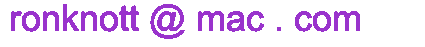updated 25 September 2016